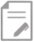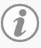Lippincott Nursing Pocket Card - February 2022

# Common Calculations## Common Calculations

Medication administration is a core competency for nurses in every clinical setting. A key skill required to safely dispense drugs is the ability to perform accurate dosage calculations. New technologies such as bar coding medication and smart infusion pumps have helped to reduce medication errors (Cookson, 2013). However, nurses cannot rely completely on these advances. Two dosage calculation techniques are presented below: traditional formulas and dimensional analysis. Nurses should select one formula and practice to become proficient in that method.

## Universal Formula (Wilson, 2013)All dosage calculations have these 2 components:

• Medication dosage prescribed by the healthcare provider
• Medication concentration supplied by the pharmacy

In the universal formula, the desired amount (D) is the dose prescribed by the provider. The amount on hand (H) is the dose on the container label. The volume (V) is the form and amount in which the drug is supplied (i.e. tablet, capsule, liquid). To use this formula, divide the desired amount by the amount on hand and multiply by the volume.

Example 1:
Administer digoxin 0.5 mg IV daily. The drug concentration available from the pharmacy is digoxin 0.25 mg/mL. How many mL will you need to administer a 0.5 mg dose?

D/H x V = Dose
0.5/0.25 x 1 = 2 mL

## Intravenous (IV) Medications (Wilson, 2013)

Continuous IV drip calculations are more complex. Use the universal formula, recognizing that some conversions are usually necessary. First, determine the drug concentration. Then consider the unit in which your drug is measured (units/hour, mg/hour, mg/min, mcg/min, or mcg/kg/minute). Then, depending on how the drug is ordered, use one of the formulas below.

To find mcg/min:If your amount on hand is in milligrams (mg), convert mg to micrograms (mcg) by multiplying by 1,000. Also, convert hours to minutes.

Example 2:
A patient is on a nitroglycerin drip. The IV pump is running at 8 mL/hour. The label on the bottle reads 50 mg in 500 mL 0.9% sodium chloride solution. What dose of nitroglycerin (mcg/min) is the patient receiving?

50 mg/500 mL x 1000 mcg/1 mg x 8 mL/1 hr x 1 hr/60 min

Most institutions utilize infusion pumps that can be programmed to the tenth or hundredth decimal place. If your institution does not have infusion pumps with this capability, you may need to round to the nearest whole number.

To find mcg/kg/min:
Use the formula for mcg/min and divide by patient’s weight (kg).Example 3: Administer dopamine at 10 mcg/kg/min. The pharmacy provides dopamine 800 mg in 250 mL of D5W. What is the hourly IV pump rate? The patient weighs 85.3 kg.

In this example, solve for mL/hr.
800 mg/250 mL x 1000 mcg/1mg x mL/hr  x 1 hr/60 min ÷  85.3 kg = 10 mcg/kg/min
800,000 mcg/250 mL x mL/hr x 1 hr/60 min ÷  85.3 kg = 10 mcg/kg/min
3,200 mcg/mL x mL/hr x 1 hr/60 min ÷  85.3 kg = 10 mcg/kg/min
3,200 mcg/mL x mL/hr x 1 hr/60 min = 853 mcg/min
mL/hr = 853 mcg/min x 1 mL/3,200 mcg x 60 min/hr
mL/hr = 16 mL/hr

To find units/hour:
First, determine the concentration of the amount on hand. Then, use the universal formula to calculate the rate.

Example 4:
Administer heparin 500 units per hour I.V. The pharmacy supplies the heparin infusion as 20,000 units in 500 mL D5W.

Find the concentration:
20,000 units/500 mL = 40 units/mL

Use the universal formula:
D/H x V = Dose
500 units/hr ÷ 40 units/mL = 12.5 mL/hour## Dimensional Analysis (Cookson, 2013)

Dimensional Analysis (DA) or factor-label method: uses a series of conversion factors of equivalency from one system of measurement to another but doesn’t require memorizing specific formulas (Koharchik & Hardy, 2013). This method reduces errors and can be used for all dosage calculations.

1. Start with the labels needed in the answer to determine what unit of measure is needed to begin setting up the calculation.
2. Build the calculation by placing information with the same label as the preceding denominator into the equation in the numerator to cancel out the unwanted labels. Repeat until all units of measure not needed in the answer are cancelled out.
3. Calculate to determine the correctly labeled numeric answer. Don’t round any numbers in the equation until you have the final answer.

Using the same examples above, let’s use the dimensional analysis method.

Example 1:
Administer digoxin 0.5 mg IV daily. The drug concentration available from the pharmacy is digoxin 0.25 mg/mL. How many mL will you need to administer a 0.5 mg dose?

Step 1: What unit of measure (label) is needed? Place this on the left side of the equation.
mL? =

Step 2: On the right side, place the information given with the same label needed in the numerator.
mL?  =        mL
0.25 mg

Step 3: Place information with the same label as the preceding denominator into the equation in the numerator to cancel out the unwanted labels. Repeat this step sequentially until all unwanted labels are canceled out.

mL?     mL          x  0.5 mg
0.25 mg

Step 4. Multiply numbers across the numerator, then multiply all the numbers across the denominator. Divide the numerator by the denominator for the final answer with the correct label.

mL? =     mL      x  0.5 mg  =   0.5 mL = 2 mL
0.25 mg                         0.25

Example 2: A patient is on a nitroglycerin drip. The pump is running at 8 mL/hour. The label on the bottle reads 50 mg in 500 mL 0.9% sodium chloride solution. What dose of nitroglycerin (mcg/min) is the patient receiving?

##### Step 1: ? mcg                min Step 2: *Convert 50 mg to 50,000 mcg.            ? mcg  =  50,000 mcg  =  100 mcg              min            500 mL             mL Step 3: ? mcg  = 100 mcg    x   8 mL   x   1 hour               min           mL              hour       60 min Step 4: ? mcg  =  100 mcg   x    8 mL   x   1 hour   =   800 mcg  =  13.3 mcg/min               min            mL             hour        60 min        60 min

Example 3: Administer dopamine at 10 mcg/kg/min. The pharmacy provides dopamine 800 mg in 250 mL of D5W. What is the hourly IV pump rate? The patient weighs 85.3 kg.

Step 1:  ? mL
hr

Step 2:  ? mL   =    250 mL
hr            800 mg

Step 3:  ? mL   =   250 mL   x     1 mg       x    10 mcg     x   85.3 kg   x     60 min
hr          800 mg      1,000 mcg        kg/min                                1 hr

Step 4: ? mL   =   250 mL   x      1 mg     x   10 mcg   x  85.3 kg  x  60 min  =  12,795,000
hr           800 mg     1,000 mcg       kg/min                          1 hr          800,000

16 mL/hr

Example 4: Administer heparin 500 units per hour I.V. The pharmacy supplies the heparin infusion as 20,000 units in 500 mL D5W.

Step 1: ? mL
hr

Step 2: ? mL   =   1 mL
hr         40 units

Step 3: ? mL   =    1 mL     x   500 units
hr          40 units             hr

Step 4: ? mL  =   500 mL  =   12.5 mL/hr
hr           40 hr

## Calculating Drops Per Minute (Koharchik & Hardy, 2013)

Continuous IV infusions are delivered via infusion pumps. While continuous IV infusions are typically delivered via infusion pumps, there may be times when an electronic pump is not available. This requires calculating the number of drops per minute, as administered through basic IV tubing.

It is important to note that there are two types of IV tubing that will deliver fluid at a specific flow rate, known as the drip factor: macrodrip and microdrip. The drip factor, which can be found printed on the IV tubing package, is the number of drops (gtts) in one mililiter (mL) of solution delivered by gravity. The rate is measured by counting the number of drops that fall into the drip chamber each minute.

• Macrodrip tubing is wider, producing larger drops and is available in three sizes: 10, 15, or 20   drops per mL (gtt/mL). Macrodrip tubing is typically used to infuse fluids at a rapid rate.
• Microdrip tubing is narrower, producing smaller drops with a drip factor of 60 gtts/mL. It is often used to infuse fluids to pediatric and neonatal patients or medications requiring a precise flow rate.

Example:
Administer lactated ringer’s solution IV at 75 mL/hour. The drip factor is 10 drops/mL. How many drops per minute will you run the infusion?

Using the DA method:
Step 1: ? drops
min

Step 2: ? drops  =  10 drops
min             mL

Step 3: ? drops   =   10 drops  75 mL   x   1 hr
min                mL              hr          60 min

Step 4: ? drops   =   10 drops    x   75 mL   x     1 hr     =   750 drops   =   12.5 or 13 drops/min
Mn                 mL               hr             60 min          60 min

To simplify, take the infusion rate and divide by the following based on the size of the tubing set.
Macrodrip

• 10 gtt/mL - divide the infusion rate by 6
• 15 gtt/mL - divide the infusion rate by 4
• 20 gtt/mL - divide the infusion rate by 3
Microdrip
• 60 gtt/mL divided by 60 is 1. Therefore, the drip rate is the same as the flow rate (drip rate = flow rate).

## General Calculation Tips:

• Have a colleague or pharmacist check your work.
• Know general therapeutic drug dose ranges for common medications.

## Dosage Calculation Conversions

 1 kilogram 1,000 grams 2.2 pounds 1 pound 0.45kg 16 ounces 1 gram 1,000 mg 15-16 grains 1 mg 1,000 mcg 1 grain 60 mg 1 liter 1,000 mL 1 quart 2 pints 4 cups 32 ounces 1 teaspoon 5 mL 60 drops 1 tablespoon 3 teaspoons 15 mL 1 ounce 2 tablespoons 30 mL 1 cup 1/2 pint 8 ounces 240-250 mL 1 pint 2 cups 16 ounces 480 mL 1 quart 2 pints 4 cups 32 ounces 1 liter 1,000 mL 1 gallon 4 quarts 8 pints 16 cups 128 ounces 3,785 mL

Temperature Conversion:
T°C = (T°F – 32) x 5/9
T°C = (T°F – 32)/1.8

###### References: Cookson K. L. (2013). Dimensional analysis: calculate dosages the easy way. Nursing, 43(6), 57–62. https://doi.org/10.1097/01.NURSE.0000428696.87216.e1 Koharchik, L.S. & Hardy, E.C. (2013). As easy as 1, 2, 3! Dosage calculations. Nursing Made Incredibly Easy!, 11(1), 25-29. https://www.doi.org/10.1097/01.NME.0000424170.34092.7a Wilson, K.M. (2013). The nurse’s quick guide to I.V. drug calculations. Nursing Made Incredibly Easy! 11(2), 1-2. https://www.doi.org/10.1097/01.NME.0000426306.10980.65